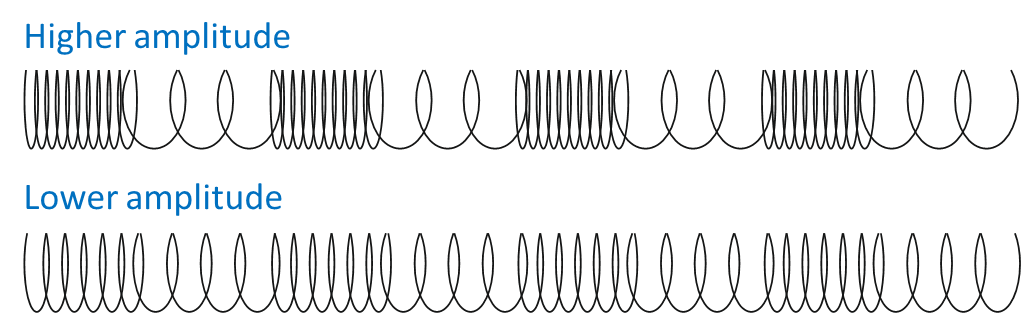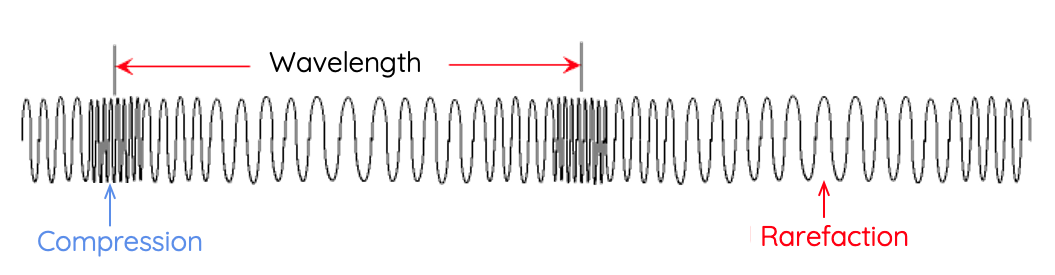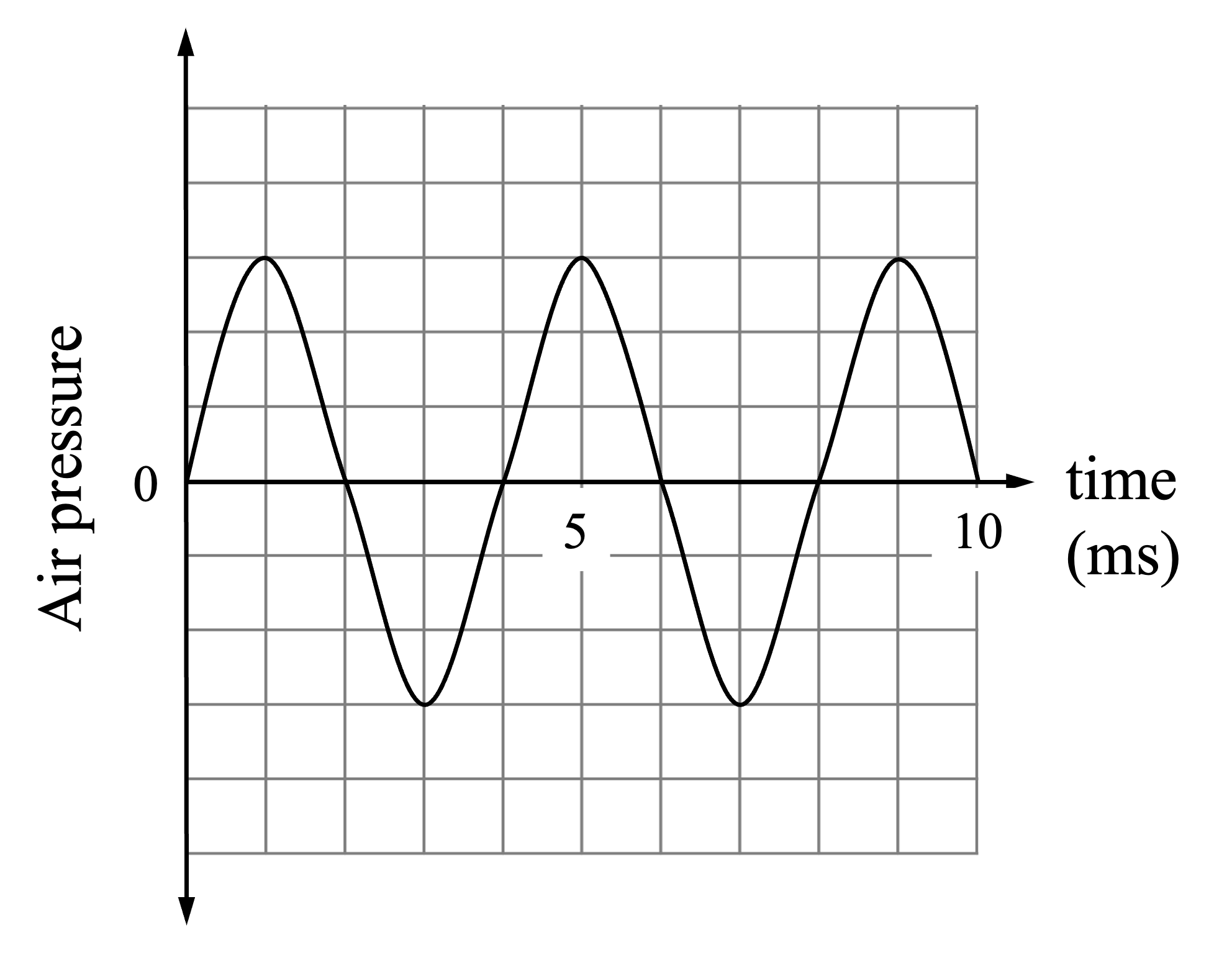# Properties of Longitudinal Waves

This topic is part of the HSC Physics course under the section Properties of Waves.

### HSC Physics Syllabus

• construct and/or interpret graphs of displacement as a function of time and as a function of position of transverse and longitudinal waves, and relate the features of those graphs to the following wave characteristics:

– velocity
– frequency
– period
– wavelength
– displacement and amplitude (ACSPH069)
• solve problems and/or make predictions by modelling and applying the following relationships to a variety of situations:

– v = f\lambda
– f = 1/T

### An Overview of Waves in HSC Physics

In the HSC Physics syllabus, understanding waves is fundamental, and their properties form the bedrock for many complex principles. In this article, we'll delve into the key concepts of waves including frequency, period, wavelength, amplitude, and velocity—tailored specifically for HSC physics students.

This article discusses the properties of transverse waves only. Properties of longitudinal waves are discussed separately here.

### What is a Longitudinal Wave?

A longitudinal wave is one where its direction of oscillation is parallel to the direction of its propagation. Longitudinal waves are all mechanical waves which require a medium to propagate.

Examples of transverse wave include the oscillation of a slinky, and sound.

### Amplitude

In transverse waves, the amplitude is the measure of the wave's maximum displacement from its equilibrium or rest position. In contrast, in longitudinal waves, the amplitude is related to the density of the medium as a wave propagates through it.

Specifically, amplitude is the maximum density caused by the disturbance by a longitudinal wave.

You can also understand amplitude as the maximum difference in density created by the oscillation of particles within a medium.In the context of sound waves, for example, the amplitude is related to the pressure variation - the difference between the maximum pressure caused by the wave's compression (when particles are bunched together) and the pressure at equilibrium.

The changes in pressure of a sound wave over a certain distance can be visualised as a sinusoidal curve (this is not suggesting that sound wave is a transverse wave). On this curve, the maximum points correspond to the points at which pressure is at its maximum. The amplitude refers to the difference between these maximum points and the equilibrium.Diagram: two longitudinal waves in slinkies with different amplitudes.

Visually, amplitude of a longitudinal wave is related to the closeness of the slinky, or how compressed it becomes during the propagation of the wave. A higher amplitude wave causes the slinky to reach a greater compression as shown on the diagram above.

The amplitude of a longitudinal wave can be challenging to determine visually because it's not as evident as the peak or trough in a transverse wave. However, it can be calculated using various methods, such as by measuring the maximum pressure variation (in sound waves) or by using specific experimental or electronic equipment. Remember, the larger the amplitude, the more energy the wave carries. In sound waves, a greater amplitude results in a louder sound.

A high amplitude wave carries more energy than a low amplitude wave. This fundamental concept applies to longitudinal waves such a sound where its amplitude relates to the volume of sound. Sound waves with higher amplitude have greater volume (louder).

### Wavelength

In HSC Physics, wavelength (λ) is a critical quantity that represents the distance taken for a wave’s shape to repeat. The SI unit for wavelength is the same as distance, metre (m). In longitudinal waves, this can be thought of as the length between two consecutive rarefactions or two consecutive compressions.

Compression refers to the areas where the particles in the medium are close together. On the other hand, rarefaction represents areas where the particles are spread apart. For gaseous media like air, compressions are associated with high pressure, while rarefactions are associated with low pressure.

The wavelength of a longitudinal wave is usually determined by the distance between two consecutive compressions or two consecutive rarefactions.One way to visualise this is by using a slinky. If you create a wave within the slinky, the parts where the coils are close together represent compressions, and the parts where the coils are spread out represent rarefactions. The distance between two successive compressions (or two successive rarefactions) represents the wavelength of the wave.

### What is Frequency?

Frequency is a measure of how many wave cycles or wavelengths occur in a unit of time. More commonly, it is defined as the number of wavelengths of a wave that pass by in one second.

In physics, frequency has the unit of Hertz (Hz), which is equal to one cycle per second.

Like amplitude, frequency of longitudinal waves can be difficult to visualise. This is made easier by translating the wave onto a two-dimensional axis where the x-axis is time and y-axis is the density of the medium throughout the wave propagates.

For example, for sound waves, air pressure can be graphed against time as shown by the following diagram.By translating the longitudinal wave onto a graph, it becomes easier to identify one wavelength, and thus the frequency of the wave (number of wavelengths in one second).

### Period

The period of a wave, often denoted by the symbol 'T', is the time take for one wavelength of a wave to pass by a certain point.

Period is given by the reciprocal of frequency:

$$T = \frac{1}{f}$$

This means that the period is the amount of time it takes for one full wave cycle to occur. For example, if a wave has a frequency of 5 Hz, its period would be 1/5, or 0.2 seconds.

Frequency and period of a wave are inversely proportional. This means as the frequency of a wave increases, its period decreases.

### Wave Velocity

Lastly, the velocity of a wave is the speed at which a wave travels.

Velocity of a wave is given by the product of its frequency and wavelength:

$$v = f \lambda$$

where

• v is the velocity in m/s
• f is the frequency in Hz or per second
• \lambda is the wavelength in metre

This equation should make sense - wavelength is the distance completed by the wave in one cycle of oscillation, and frequency is the number of wavelength passing by per second. When multiplied together, the result represents the distance travelled by the wave per second, which is its speed.

Velocities vary between waves and the media

Wave velocity depends on the medium through which the wave is traveling. This concept explains why light, for instance, travels at different speeds through air, water, or glass.

Sound waves travel faster in solids, e.g. metals, and slower in gases e.g. air.

When a wave's velocity is kept constant, its frequency is inversely proportional to its wavelength. This means as its frequency increases, its wavelength becomes smaller.

### Example 1

Given that the speed of sound in air is 340 m/s, what is the wavelength of the sound wave if it has a frequency of 800 Hz?

Solution:

$$v = f \lambda$$

$$\lambda = \frac{v}{f}$$

$$\lambda = \frac{340}{800}$$

$$\lambda = 0.425 \, \text{m}$$

### Example 2

The graph shows the changes in air pressure as a sound wave is projected through a hollow tube.(a) Identify the time points at which compressions and rarefactions occur.

(b) Given that the velocity of sound in air is 340 m/s, calculate the wavelength of this sound wave.

Solution:

(a) Compressions correspond to maximum air pressure, which occurs at t = 1, 5 and 9 milliseconds. Rarefactions correspond to maximum air pressure, which occurs at = 3 and 7 milliseconds.

(b) First, we can find the period from the graph, T = 4 s.

$$f = \frac{1}{T}$$

$$f = \frac{1}{4 \times 10^{-3}} = 250 \, \text{Hz}$$

Using the frequency and velocity, we then calculate the wavelength:

$$v = f \lambda$$

$$\lambda = \frac{v}{f} = \frac{340}{250} = 1.36 \, \text{m}$$